Cayley Sextic
CAYLEY SEXTIC

Balmoral Software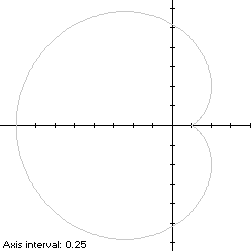The Cayley sextic having its cusp on the right is a heart-shaped closed curve S with polar equation
r(t) = -2cos3(t/3), -pi ≤ t < pi
For a simple curve describing the outer envelope of the Cayley sextic, T is restricted to the interval [-π,π]. The scaling factor -2 is used for comparison with the cardioid. Since r(t) is an even function of t, the Cayley sextic is symmetric with respect to the x-axis.

The curve is traced out in a counterclockwise direction, starting from the cusp (1/4,0) at t = -π. Its minimum abscissa occurs at the point (-2,0) when t = 0. Its maximum abscissas occur at the points (1/2,±1/2) when t = ∓3π/4, which determine that the Cayley sextic is non-convex by the multiple local extrema test. The extreme ordinates of the Cayley sextic occur at the pointswhen t = ∓3π/8, so the width x height of its bounding rectangle is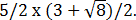### Metrics

We have
r'(t) = 2sin(t/3)cos2(t/3),
so by (L2), the perimeter of this portion of the Cayley sextic is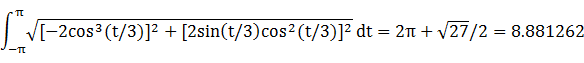By (A2), the corresponding area isBy (C2), the centroid abscissa of this portion of the Cayley sextic is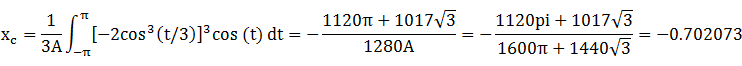The bounding rectangle for inconics is delimited by the cusp at (1/4,0).

### Convex Hull

The convex hull is created by connecting the maximum abscissa points with a vertical line segment of length 1 at x = 1/2. By (L2), the perimeter of the convex hull is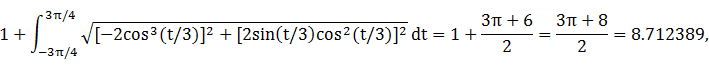which is about 2% shorter than that of the Cayley sextic portion.

The line segment of the convex hull creates an isosceles triangle with the origin, having area (1/2)2 = 1/4, as shown in blue in the left diagram below. The centroid abscissa of this triangle is the average of its vertex abscissas, or 1/3. By (A2), the remainder of the convex hull has area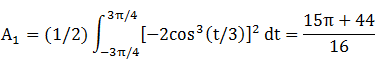By (C2), the associated centroid abscissa is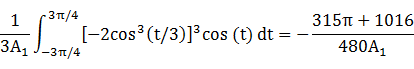The convex hull component metrics can be summarized as follows:
 Area Centroid abscissa Product Region Triangle 1/4 1/3 1/12 Remainder A1 = (15π + 44)/16 -(315π + 1016)/(480A1) -(315π + 1016)/480 Total 15π/16 + 3 -(315π + 976)/480
The area of the convex hull is about 1% larger than that of the Cayley sextic. The centroid abscissa of the convex hull is the weighted average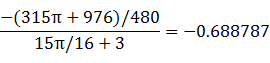### Incircle

A candidate incircle is located on the x-axis between the minimum abscissa -2 and the cusp (1/4,0), and so has radius R = 9/8 and center abscissa c = -7/8. For verification, we have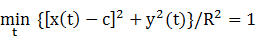### Inellipse

Using z = 1/4 in Lemma E,
d/dt [x(t) - z]y(t) = d/dt 4sin(t)cos(t)cos6(t/3) + sin(t)cos3(t/3)/2
has a zero at t* = -0.707452. The corresponding coordinates are
x* = -1.39729

y* = 1.19484

We then have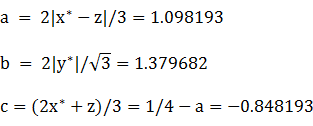For verification,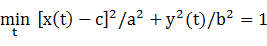### Circumellipse

Using z = -2 in Lemma E,
d/dt [x(t) - z]y(t) = d/dt 4sin(t)cos6(t/3)cos(t) - 4sin(t)cos3(t/3)
has a zero at t* = -1.598518. The corresponding coordinates are
x* = 0.035429

y* = 1.2777

We then haveFor verification,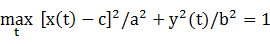### Circumcircle

The radius of a circle centered on the x-axis and circumscribing S is at least its maximum ordinate, so a candidate for the circumcircle has radius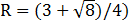and center abscissa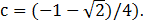For verification,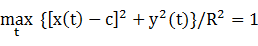### Summary Table

 Perimeter Area Centroid Figure Parameters Incircle R = 9/8 7.068584 3.976078 (-0.875,0) Inellipse a = 1.098193b = 1.379682 7.809609 4.760006 (-0.848193,0) Cayley sextic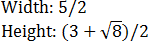8.881262 5.875548 (-0.702073,0) Convex hull 8.712389 5.945243 (-0.688787,0) Circumellipse a = 1.356954b = 1.475361 8.901868 6.289459 (-0.643046,0) Circumcircle R =9.155272 6.670104 (-0.603553,0)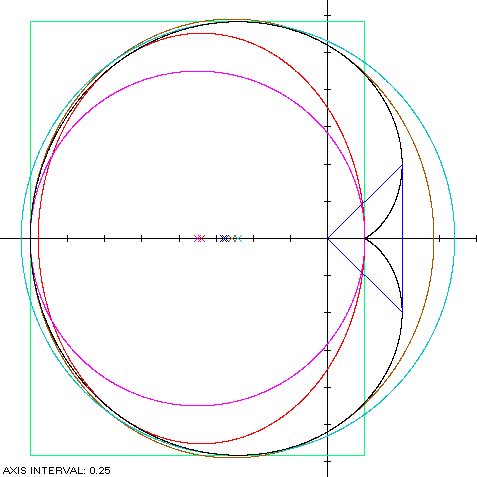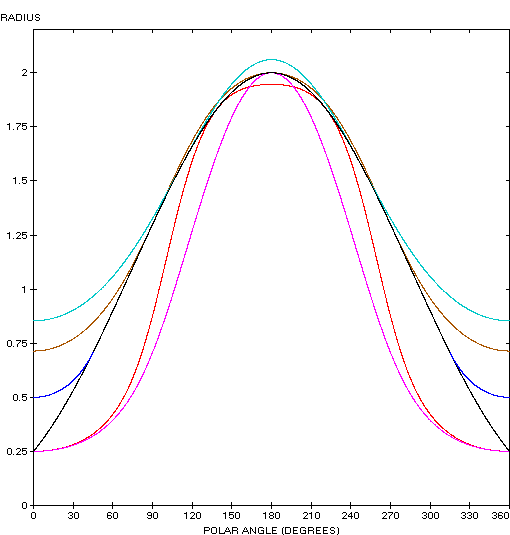The Cayley sextic (red) is a member of a group of similarly-shaped figures described on these pages, including (inside to outside) the rotated lima bean curve, the cardioid and the cochleoid: